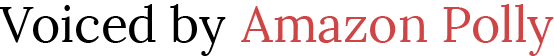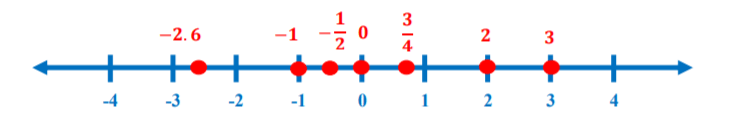# Teaching the Real Number System

 Would You Rather Listen to the Lesson?One of the first things that students learn when diving into algebra is the real number system. This entails learning about the different number sets that the real number system is composed of, such as rational and irrational numbers, integers and non-integers, negative and whole numbers…

To put it in a nutshell, the variety of number sets can sometimes baffle even the best of students! Luckily, we’re here to lend a helping hand! If you’re teaching the real number system, here are some great guidelines and activities to help you best prepare for success in your lessons!

## What Is the Real Number System?

Explain to students that the real number system is a system comprising real numbers. A real number is almost any number that you can think of. Provide several examples on the whiteboard, such as:

16, – 58, 3⁄4, 5, ½, 478, 209, 45⁄5, √7 , π

Real numbers include rational and irrational number sets. Rational numbers include non-integers and integers, whereas integers comprise whole numbers and negative numbers. Finally, whole numbers consist of positive numbers and zero.

You can draw a chart like the one on the Guided Notes Worksheet (Members Only) to help children visualize all the subsets of numbers that form real numbers. Then, define all the number sets that real numbers include.### Rational Numbers

You can define rational numbers as all the numbers that can be expressed as a ratio of two integers, in the form of a ⁄ b, where b does not equal 0. Provide a few examples and explanations of why these numbers are rational numbers, such as:

• 7 is a rational number (because we can write it as 7⁄1)
• 9 is a rational number (because we can simplify it to 3 and express 3 as 3⁄1)
• 1.5 is a rational number (because we can write it as 3⁄2)
• – 6.6 is a rational number (because we can write it as -66⁄10)
• 0.75 is a rational number (because we can write it as 3⁄4)
• 1⁄2 is a rational number (because it’s expressed as a ratio of two integers) etc.

Finally, add that the decimal representation of rational numbers is either terminating or repeating.

### Irrational Numbers

After having defined rational numbers, introduce irrational numbers. You can define them as numbers that can’t be expressed as a ratio of two integers and whose decimal representation goes on forever without repeating.

Make sure to point out that we call these irrational not because they’re ‘crazy’, but simply because they can’t be expressed as a ratio of two integers (hence, irrational). Provide a few examples of irrational numbers, such as:

π, √3, √7, √2, √1.6, 0.09009000900009…, 5⁄0, 8⁄0

### Integers and Non-Integers

Explain to students that rational numbers include integers and non-integers. Define non-integers as numbers that are neither a zero nor a whole number. This includes fractions, decimals, and imaginary numbers (see below under ‘what about complex numbers’ for more information on imaginary numbers).

Provide a few examples of non-integers, such as:

– 0.25, 3.74, 1⁄8, – 3⁄4, 9i…

Then, define what integers are. You can simply say that integers represent both positive and negative whole numbers, as well as zero. Provide a few examples of integers:

-24, 1, 9, 38, 202, – 45, 3, – 11…

You may also want to check out our article on teaching integers, as well as our article on rounding multi-digit whole numbers.Point out that complex numbers are the only numbers that don’t fall in the category of real numbers. You can define complex numbers as numbers that are of the form a + bi, where a and b represent real numbers, and i is called an imaginary unit, thus, bi is also an imaginary number.

Explain why i is imaginary. Point out that i = √-1, because  = – 1. So when squaring an imaginary number we get a negative number. Yet, we know that by squaring a positive number we get a positive number, and that by squaring a negative number we get a positive number (i.e. -2 x -2 = 4).

Explain to students that imaginary numbers were introduced because we wanted to imagine that such numbers existed, that is, numbers that can give a negative result when squared. This may come across as confusing for students in lower grades, but you can add that imaginary numbers have a great deal of usefulness in advanced physics, among other things.

Finally, present several examples of complex numbers, such as:

3 + 4i ; 8 + 9i ; 6 – 3i

### Absolute Value of Real Numbers

After students understand the different number sets in the real number system and are able to fluently differentiate them, you can introduce the concept of absolute value of real numbers. You can define the absolute value of a number as the distance of that number from zero.

Point out that we use two vertical bars to represent the absolute value of a number and provide a few examples:

|-4|, |3.2|, |7|, |51|, |- ½ |…

You can also draw a number line on the whiteboard to demonstrate absolute value. Explain that we can find the absolute value of both positive and negative numbers and illustrate this on the number line.

For instance, show students that since the distance of 9 from 0 is 9, the absolute value of 9 is 9. In other words, we can simply write |9| = 9. Then, you can explain how to find the absolute value of negative numbers.

Point out that even with negative numbers, we’ll still have a positive number as an absolute value. This is because according to the above definition, absolute value is the distance of a number from 0, so a negative number is also the distance from 0 to – 9, that is, 9 (we’ll move 9 ‘spaces’ on the number line).

Make sure to also check out our article on absolute value.

For additional guidelines on the real number system, feel free to print out this Guided Notes Worksheet (Members Only) and distribute a copy to each student.

## Activities to Practice the Real Number System

This section contains a mixture of offline and online activities that you can use in your classroom to further reinforce students’ knowledge of the real number system. For activities on absolute value, refer to this article.

### Real Numbers on the Number LineThis activity invites students to order real numbers on a number line, that is, a line where numbers are written at intervals. Students will thus practice their understanding of whether a number is bigger or smaller by arranging them in ascending order.

To implement this activity in your classroom, create several number lines (one per group, depending on the size of the class). Then, create a variety of real numbers. You can use markers in different colors to add to the visual appeal of the number line.

Divide students into groups of 3, 4. Place one number line in each group and a pile of different numbers. Make sure that the number line is big enough for the numbers you have created (you don’t want to end up with -8.23 on a number line that only goes up to -5!).

Each group works together and tries to arrange the numbers on the number line in ascending order. When they finish, they present their number line and discuss why they arranged the numbers in that particular order.

To make the activity more challenging, you can also include graphing the absolute value of real numbers, such as |-4.3|, |0.5|, |8|, |-3|, |-½|, etc.

### Classifying Numbers Game

In this simple online game, students will sharpen their real number identification skills. The game contains a circle of a larger set of the real number system and smaller circles of all the number sets inside this large circle, such as integers, whole numbers, (ir)rational numbers…

Players are presented with several questions, each question containing a few numbers that have to be placed in the appropriate circle. A player cannot move on to the next question before answering the previous question correctly, which encourages students to review mistakes.

You can implement this game in a classroom by pairing up your students. Students play simultaneously on different devices, seated in a way so that they’re unable to see each other’s answers. The first one that answers all questions is declared the winner of the game.### Rational Number Game

This cool virtual game is guaranteed to get your students excited about rational and irrational numbers! It features an awesome storyline, where scientists discover that irrational numbers can cause insanity in people.

The more cells infected with irrational numbers, the more insane this person becomes. So players are on a mission – collect all rational numbers, avoid the irrational numbers and try to destroy the infected cells.

Each student plays individually. In lack of separate devices for all students, you can also decide to pair students up.

### Rational or Irrational?

This is a fun and interactive online game that allows students to practice quick recognition of rational and irrational numbers. In classrooms, students can play it in pairs to make it more competitive. Homeschooling parents can also use it for individual practice with their children.

The game features two wooden boxes in nature and birds that are quickly flying over them. The flying birds are holding a number with their beaks. The player must quickly ‘catch’ the number and drop it in the correct box.

The game measures the score for each player, as well as the time. The game ends when a person manages to score 1,000 points, that is, to put all numbers in the correct box. So, if students are competing in pairs, the first person that manages to score 1, 000 points wins the game.

### Rags to Riches Game

This is an online game that is very similar to the ‘Who Wants to Be a Millionaire? game. Students who have already played ‘Who Wants to Be a Millionaire’ should be familiar with the rules.

Basically, students are asked to answer questions related to the real number system and its number sets, in order to check whether they understand how to correctly classify numbers according to their properties.

Students are offered multiple answers that they can choose from. If they answer correctly, they win a certain sum and move on to the next question. As the student proceeds, the difficulty of the question increases, as well as the sum that can be won.

The most that a student can win is \$1,000,000. If a student answers even one question incorrectly, the game is over and the student ends up with the sum they won until then. The game can be played individually, making it ideal for homeschooling parents, as well.

## Before You Leave…

If you liked these guidelines and activities, we have a whole lesson with worksheets and fun resources on teaching the real number system. So why not sign up to our emails for more free lessons and content!

In addition, you can sign up for a membership on MathTeacherCoach or head over to our blog, where you’ll find more math materials for kids of all ages. You’ll discover that with the resources we offer, teaching math is super fun and easy!